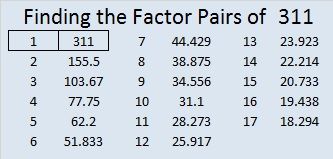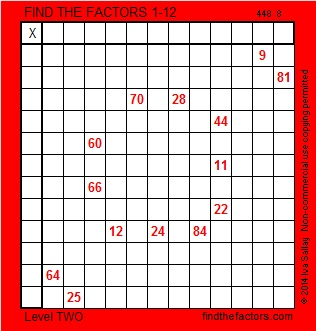# 311 and Level 2

• 311 is a prime number.
• Prime factorization: 311 is prime.
• The exponent of prime number 311 is 1. Adding 1 to that exponent we get (1 + 1) = 2. Therefore 311 has exactly 2 factors.
• Factors of 311: 1, 311
• Factor pairs: 311 = 1 x 311
• 311 has no square factors that allow its square root to be simplified. √311 ≈ 17.635How do we know that 311 is a prime number? If 311 were not a prime number, then it would be divisible by at least one prime number less than or equal to √311 ≈ 17.635. Since 311 cannot be divided evenly by 2, 3, 5, 7, 11, 13, or 17, we know that 311 is a prime number.

Here’s a little piece of red and white candy for your enjoyment:Print the puzzles or type the factors on this excel file: 12 Factors 2014-12-01This site uses Akismet to reduce spam. Learn how your comment data is processed.